# QUANTUM MECHANICS Classical mechanics is an approximation of

• Slides: 21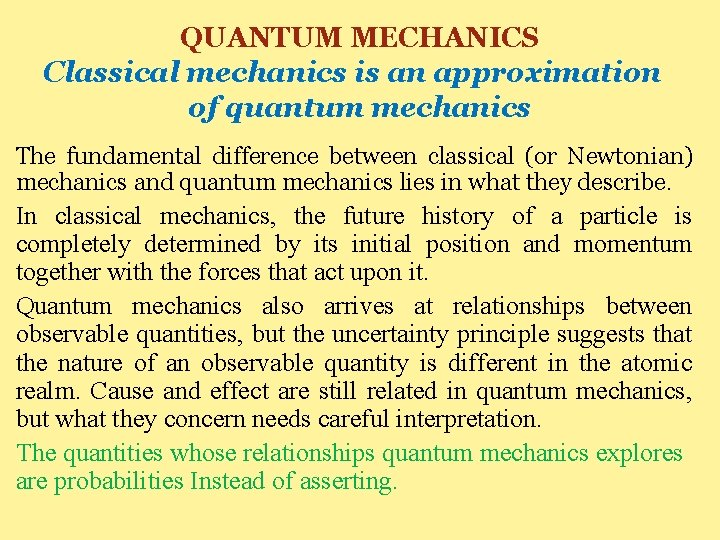QUANTUM MECHANICS Classical mechanics is an approximation of quantum mechanics The fundamental difference between classical (or Newtonian) mechanics and quantum mechanics lies in what they describe. In classical mechanics, the future history of a particle is completely determined by its initial position and momentum together with the forces that act upon it. Quantum mechanics also arrives at relationships between observable quantities, but the uncertainty principle suggests that the nature of an observable quantity is different in the atomic realm. Cause and effect are still related in quantum mechanics, but what they concern needs careful interpretation. The quantities whose relationships quantum mechanics explores are probabilities Instead of asserting.Wave Function The quantity with which quantum mechanics is concerned is the wave function Ψ of a body. While Ψ itself has no physical interpretation, the square of its absolute magnitude | Ψ | 2 evaluated at a particular place at a particular time is proportional to the probability of finding the body there at that time. 1. The linear momentum. 2. angular momentum, 3. energy of the body are other quantities that can be established from Ψ. The problem of quantum mechanics is to determine Ψ for a body when its freedom of motion is limited by the action of external forces.Wave functions are usually complex with both real and imaginary parts. A probability, however, must be a positive real quantity. The probability density |Ψ| 2 for a complex is therefore taken as the product Ψ*Ψ of and its complex conjugate Ψ*. The complex conjugate of any function is obtained by replacing i (=√-1) by –i. wherever it appears in the function. Every complex function can be written in the form: Ψ=A+i. B where A and B are real functions. The complex conjugate Ψ* of Ψ is: Ψ*=A-i. B Ψ 2= Ψ*Ψ =(A-i. B)(A+i. B)= A 2 -i 2 B 2= A 2+B 2 since i 2=-1. Hence |Ψ|2= Ψ*Ψ is always a positive real quantity, as required.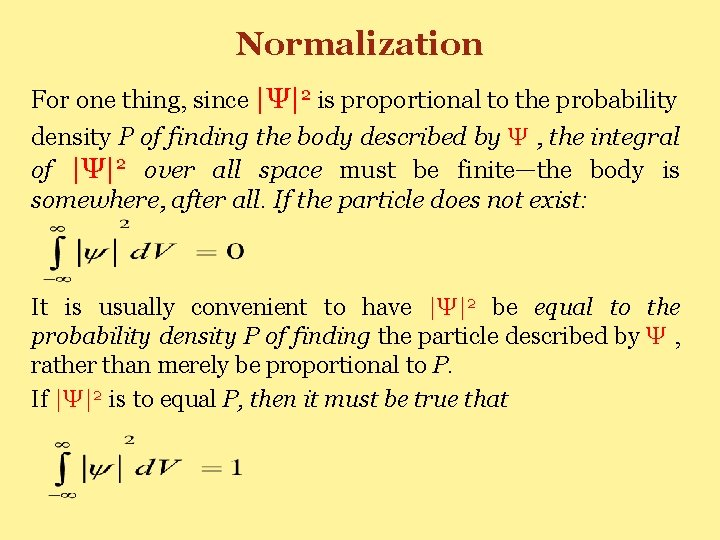Normalization For one thing, since |Ψ|2 is proportional to the probability density P of finding the body described by Ψ , the integral of |Ψ|2 over all space must be finite—the body is somewhere, after all. If the particle does not exist: It is usually convenient to have |Ψ|2 be equal to the probability density P of finding the particle described by Ψ , rather than merely be proportional to P. If |Ψ|2 is to equal P, then it must be true that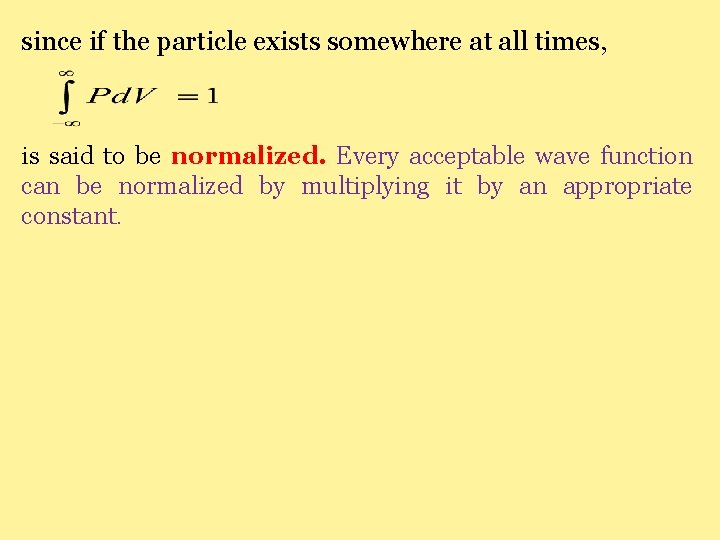since if the particle exists somewhere at all times, is said to be normalized. Every acceptable wave function can be normalized by multiplying it by an appropriate constant.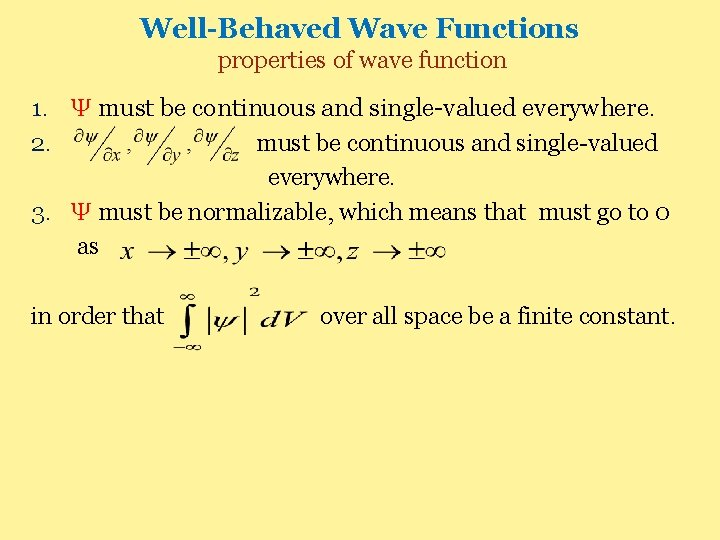Well-Behaved Wave Functions properties of wave function 1. Ψ must be continuous and single-valued everywhere. 2. must be continuous and single-valued everywhere. 3. Ψ must be normalizable, which means that must go to 0 as in order that over all space be a finite constant.THE WAVE EQUATION It can have a variety of solutions, including complex ones the wave equation is : which governs a wave whose variable quantity is y that propagates in the x direction with the speed ʋ. In the case of a wave in a stretched string, y is the displacement of the string from the x axis; in the case of a sound wave, y is the pressure difference; in the case of a light wave, y is either the electric or the magnetic field magnitude. Equation (5. 3) can be derived from the second law of motion for mechanical waves and from Maxwell’s equations for electromagnetic waves.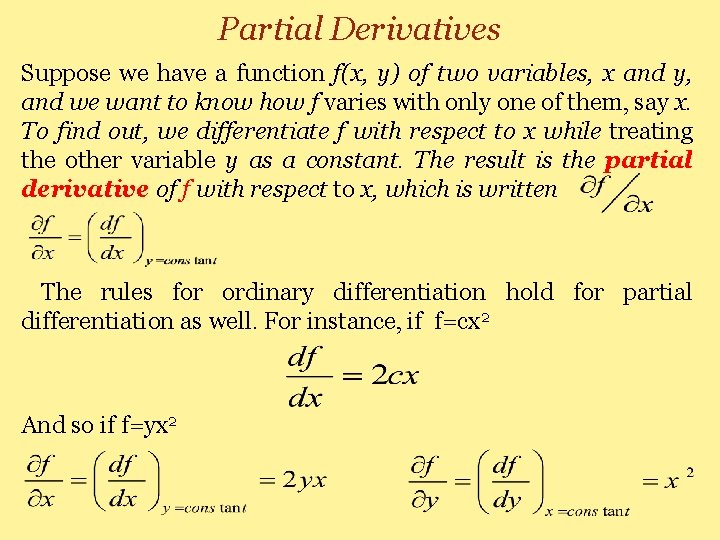Partial Derivatives Suppose we have a function f(x, y) of two variables, x and y, and we want to know how f varies with only one of them, say x. To find out, we differentiate f with respect to x while treating the other variable y as a constant. The result is the partial derivative of f with respect to x, which is written The rules for ordinary differentiation hold for partial differentiation as well. For instance, if f=cx 2 And so if f=yx 2Second order partial derivatives occur often in physics, as in the wave equation. To find , we first calculate and then differentiate again, still keeping y constant: for f=yx 2 Similarly Solutions of the wave equation may be of many kindsa standing wave in a string fastened at both ends, and so on. All solutions must be of the form where F is any function that can be differentiated. The solutions represent waves traveling in the +x direction, and the solutions waves traveling in the -x direction. represent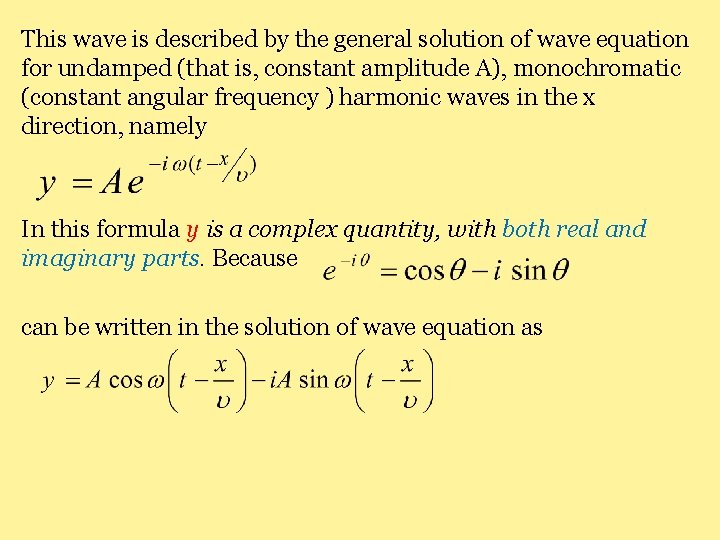This wave is described by the general solution of wave equation for undamped (that is, constant amplitude A), monochromatic (constant angular frequency ) harmonic waves in the x direction, namely In this formula y is a complex quantity, with both real and imaginary parts. Because can be written in the solution of wave equation as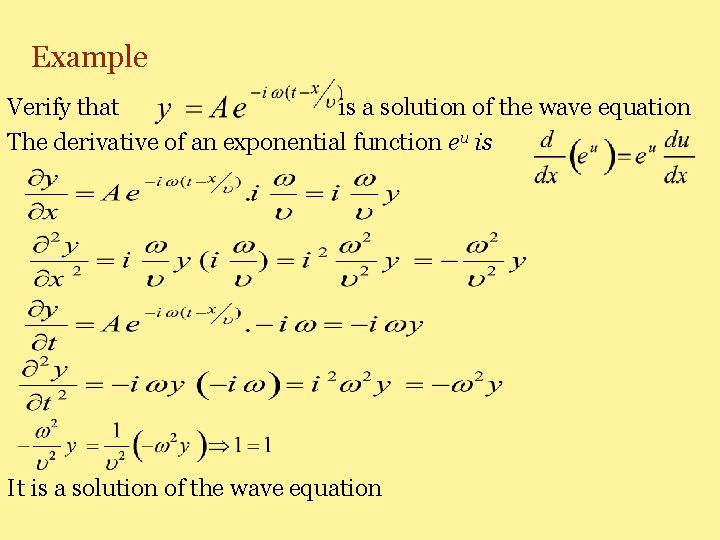Example Verify that is a solution of the wave equation The derivative of an exponential function eu is It is a solution of the wave equation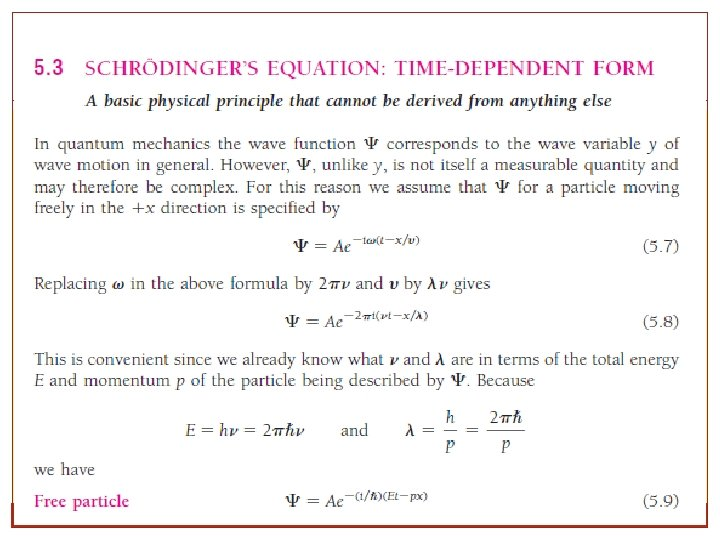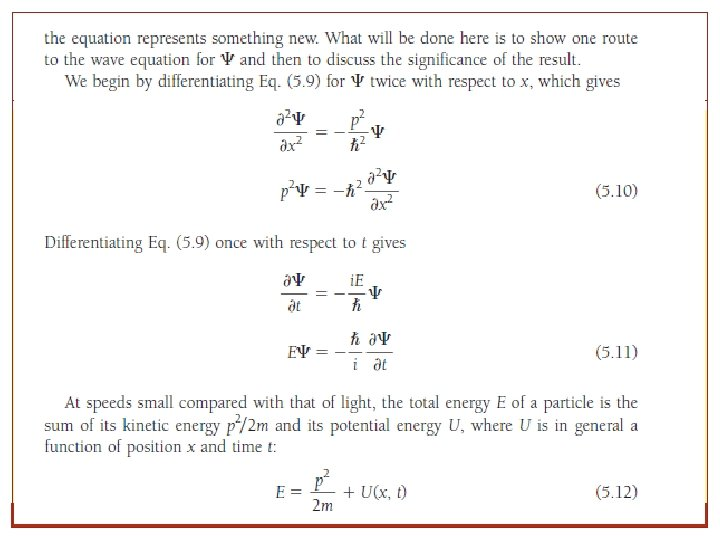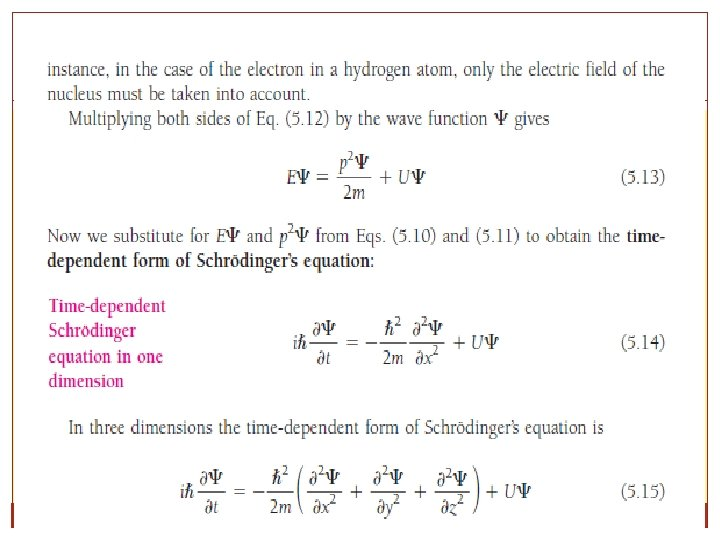SCHRÖDINGER’S EQUATION: TIMEDEPENDENT FORM In quantum mechanics the wave function Ψ corresponds to the wave variable y of wave motion in general. in one dimension is Time-dependent Schrödinger equation Time-independent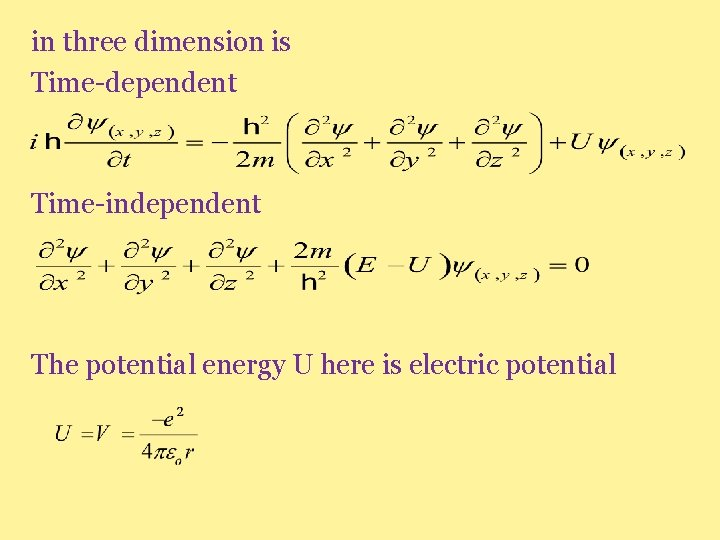in three dimension is Time-dependent Time-independent The potential energy U here is electric potential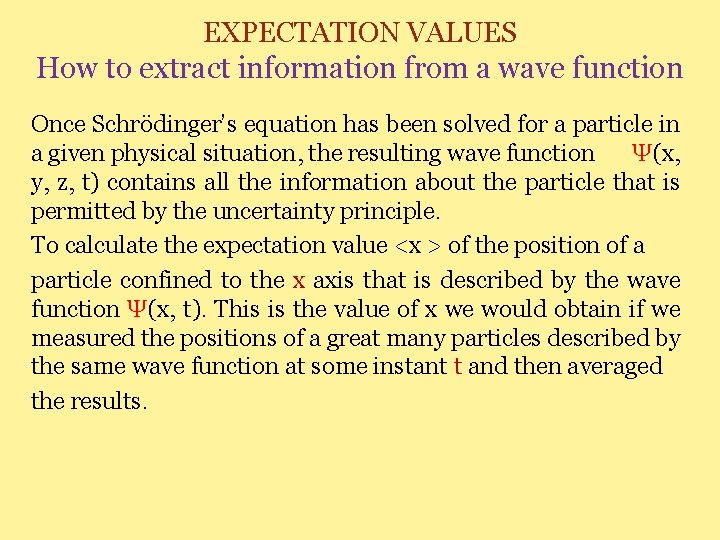EXPECTATION VALUES How to extract information from a wave function Once Schrödinger’s equation has been solved for a particle in a given physical situation, the resulting wave function Ψ(x, y, z, t) contains all the information about the particle that is permitted by the uncertainty principle. To calculate the expectation value <x > of the position of a particle confined to the x axis that is described by the wave function Ψ(x, t). This is the value of x we would obtain if we measured the positions of a great many particles described by the same wave function at some instant t and then averaged the results.we see that the expectation value of the position of the single particle is: If Ψ is a normalized wave function, the denominator of equation equals the probability that the particle exists somewhere between x =- and x = and therefore has the value 1 In this case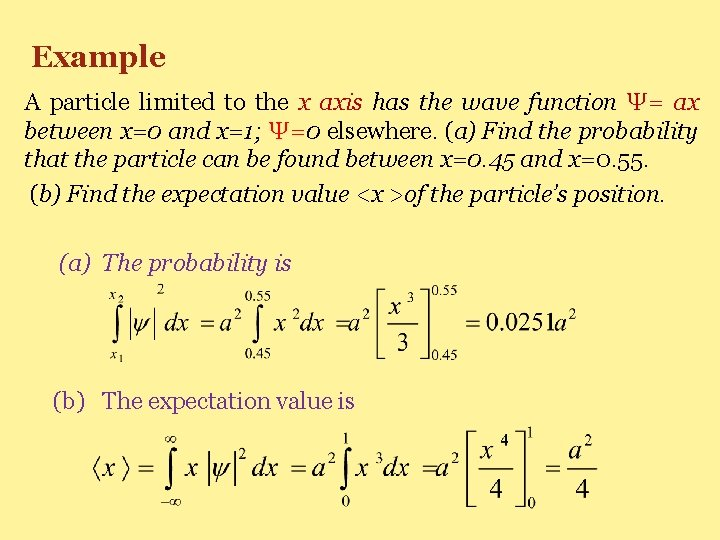Example A particle limited to the x axis has the wave function Ψ= ax between x=0 and x=1; Ψ=0 elsewhere. (a) Find the probability that the particle can be found between x=0. 45 and x=0. 55. (b) Find the expectation value <x >of the particle’s position. (a) The probability is (b) The expectation value is